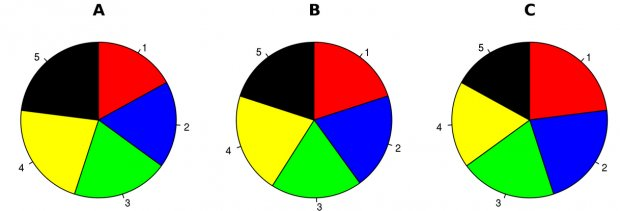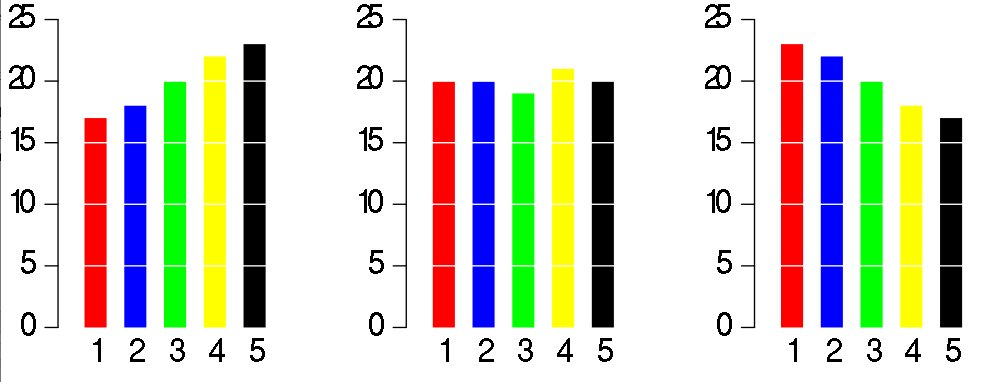# Chapter 2

## Summarizing Data

### Learning Outcomes

• Use appropriate visualizations for different types of data (e.g. histogram, barplot, scatterplot, boxplot, etc.).
• Use different measures of center and spread and be able to describe the robustness of different statistics.
• Describe the shape of distributions vis-a-vis histograms and boxplots.
• Create and intepret contingency and frequency tables (one- and two-way tables).

### Videos

Summarizing and Graphing Numerical Data

Exploring Categorical Data

There is only one pie chart in OpenIntro Statistics (Diez, Barr, & ??etinkaya-Rundel, 2015, p. 48). Consider the following three pie charts that represent the preference of five different colors. Is there a difference between the three pie charts? This is probably a difficult to answer.However, consider the bar plot below. Here, we cleary see there is a difference between the ratio of the three colors. As John Tukey famously said:

There is no data that can be displayed in a pie chart that cannot better be displayed in some other type of chartLast updated on Sat Apr 29, 2017
Published on Sat Apr 29, 2017
Edit on GitHub